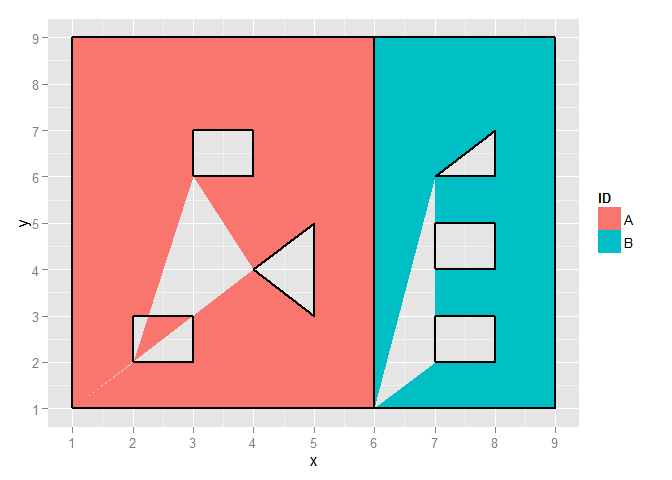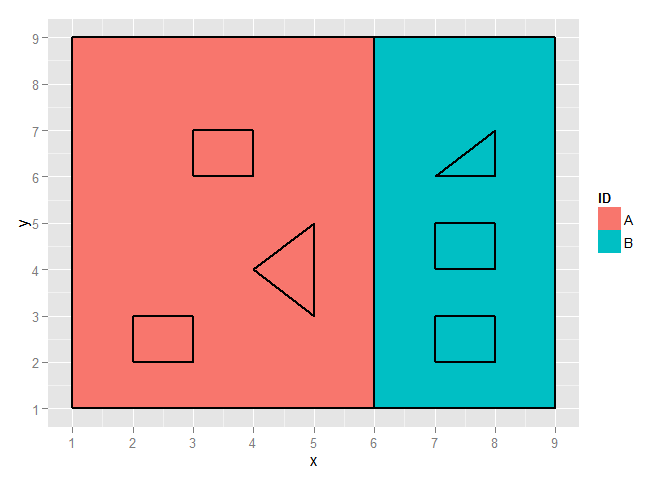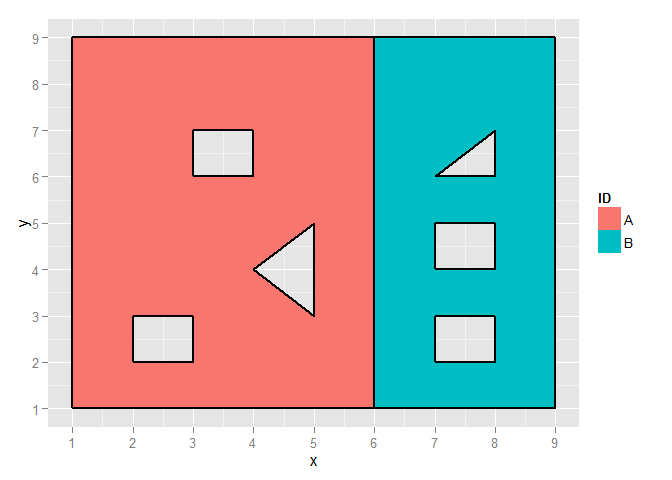Have you ever noticed that ggplot2 and polygon holes don’t play nicely. Here is an example. Say you have a data.frame containing polygons which contain holes.

require(ggplot2)
## Loading required package: ggplot2
## Warning: package 'ggplot2' was built under R version 3.1.2
#set up the data frame.  The outer ring is defined in an anti-clockwise direction and the
#inner holes clockwise.  I have also labelled the holes.

pos <- data.frame(
id =toupper(letters[
#parent     hole1      hole2      hole3    parent     hole1      hole2      hole3
c(1,1,1,1,1, 1,1,1,1,1, 1,1,1,1,1, 1,1,1,1, 2,2,2,2,2, 2,2,2,2,2, 2,2,2,2,2, 2,2,2,2)]),
ring = c(1,1,1,1,1, 2,2,2,2,2, 3,3,3,3,3, 4,4,4,4, 5,5,5,5,5, 6,6,6,6,6, 7,7,7,7,7, 8,8,8,8),
x = c(1,6,6,1,1, 2,2,3,3,2, 3,3,4,4,3, 4,5,5,4, 6,9,9,6,6, 7,8,8,7,7, 7,8,8,7,7, 7,8,8,7),
y = c(1,1,9,9,1, 2,3,3,2,2, 6,7,7,6,6, 4,5,3,4, 1,1,9,9,1, 2,2,3,3,2, 4,4,5,5,4, 6,7,6,6),
hole = c(F,F,F,F,F, T,T,T,T,T, T,T,T,T,T, T,T,T,T, F,F,F,F,F, T,T,T,T,T, T,T,T,T,T, T,T,T,T))

# Plot polygons based on the $id and fill in the borders based on the$ring
gg1 <- ggplot(pos, aes(x=x, y=y)) +
geom_polygon(aes(group=id, fill=factor(id))) +
geom_path(aes(group=ring),size=1) +
scale_x_continuous(breaks=1:10)+
scale_y_continuous(breaks=1:10)+
scale_fill_discrete("ID")
print(gg1)The borders are okay, but the polygons are mangled as the jump from the end of the outside ring and then from hole to hole carves out another polygon which is excluded from the fill.

If we use the group parameter to specify the ring value instead of the id value, then the plot looks like this.

gg2 <- ggplot(pos, aes(x=x, y=y)) +
geom_polygon(aes(group=ring, fill=factor(id))) +
geom_path(aes(group=ring),size=1) +
scale_x_continuous(breaks=1:10)+
scale_y_continuous(breaks=1:10)+
scale_fill_discrete("ID")
print(gg2)The borders are okay and the polygons are okay, it is just that they are no longer holes. ggplot2 has ignored the fact that the inner holes are defined in a closkwise direction.

So lets fix the polygons. First define a function that when passed an xy matrix of points finds the closest row in that matrix to the first row of the matrix. I want to join the holes together in a way that does not result in a line crossing another hole. So my logic is that joining the closest shapes at their closest points will avoid this happenning. The logic will still fail if the join line passes outside the perimeter of the outer shape, but how often does that happen !

closest_to_first_row <- function (xy){
if (nrow(xy)==2){
return (2)
} else {
return(1 + which.min(rowSums((xy[-1,] - xy[1,])^2)))
}
}

Now define a function that when passed two xy boundary matrices finds the closest points between the perimeters of the two rings. I don’t test for it but they have to be rings.

bridge <- function(xy1, xy2){
#which row in xy2 is closeset to mid point of xy1
i.2 <- which.min(apply((colMeans(apply(xy1, 2,range))-xy2)^2,1,sum))
i.1 <- which.min(apply((xy2[i.2,]-xy1)^2,1,sum)) #which row in xy1 is closest to this point in xy2
i.2 <- which.min(apply((xy1[i.1,]-xy2)^2,1,sum)) #and again
return(as.integer(c(i.1,i.2)))
}

Now all I need to do is bridge all the holes within a polygon to each other with a bridge of width zero. I need to calculate some summaries of the ring structures which could be done in plyr but I prefer data.table.

require(data.table)
## Loading required package: data.table
pos<-as.data.table(pos)

#Add a new field to contain the original position as I need this for
#drawing the orginal borders (which are correct)
pos$draw=pos$ring

while(TRUE){
#summarise the information on each ring
holes <- pos[hole==TRUE,.(mid.x=mean(range(x)), mid.y=mean(range(y))),by=.(id,ring)]
holes <- holes[,num_holes:=.N ,by=id]
if(max(holes[,num_holes])<=1) break  #Exit if only one hole per id remaining

#get the first id that has more than one hole in it
ii <- which(holes$num_holes>1) h.id <- holes$id[ii]                  #which id are we dealing with
h1.ring <- holes$ring[ii] #which hole are we dealing with first #get the hole ring which is closest to h1.ring This ensures that the shortest path #between h1.ring and h2.ring does not cross another hole ring. h2.ring <- holes[id==h.id][closest_to_first_row(as.matrix(holes[id==h.id, .(mid.x,mid.y)])),ring] cat('joining ring', h1.ring, 'to ring', h2.ring, '\n') #find the best bridging point h1.xy <- as.matrix(pos[id==h.id & ring==h1.ring, .(x, y)]) #xy matrix for ring1 h2.xy <- as.matrix(pos[id==h.id & ring==h2.ring, .(x, y)]) #xy matrix for ring2 h1.l <- nrow(h1.xy) #number of points in ring1 h2.l <- nrow(h2.xy) #number of points in ring2 h1.draw <- pos[id==h.id & ring==h1.ring, draw] #existing values for drawing border h2.draw <- pos[id==h.id & ring==h2.ring, draw] b <- bridge(h1.xy, h2.xy) #b is the row in h1 and b is the row in h2 to bridge #reorder h2 values about the bridging point and insert into the bridge point in h1 new.xy <- rbind( h1.xy[seq(b),] #h1 points up to the bridge ,h2.xy[seq(b, h2.l-1),] #h2 from over the bridge to one before the tail=head ,h2.xy[seq(1,b),] #h2 from the head to the bridge again ,h1.xy[seq(b, h1.l),] #h1 from the bridge to the tail ) new.draw <- c( h1.draw[seq(b)] #arrange the 'draw' to line up with the orginal rings ,h2.draw[seq(b, h2.l-1)] #so can jump from one ring to another without drawing ,h2.draw[seq(1,b)] #a border over the jump ,h1.draw[seq(b, h1.l)] ) #delete the old values and replace with the new values drop.rows <- which(pos$id==h.id & (pos$ring==h1.ring|pos$ring==h2.ring))

#update the pos data frame by dropping the original and adding the new
pos <- rbind(pos[-drop.rows,]
,data.frame(id=h.id
,ring=h1.ring
,x=new.xy[,1]
,y=new.xy[,2]
,hole=TRUE
,draw=new.draw)
)
}
## joining ring 2 to ring 4
## joining ring 3 to ring 2
## joining ring 6 to ring 7
## joining ring 8 to ring 6
#reorder the pos data.frame according to the new rings (with the holes merged)
pos<-pos[order(id,ring),]

So now lets look at the plot. Each time we jump from an original ring to another original ring the border drawing gets switched off by using group=draw.

gg3 <- ggplot(pos, aes(x=x, y=y)) +
geom_polygon(aes(group=id, fill=factor(id))) +
geom_path(data=pos, aes(x=x, y=y, group=draw),size=1) +  #draw is the original ring
scale_x_continuous(breaks=1:10)+
scale_y_continuous(breaks=1:10)+
scale_fill_discrete("ID")

print(gg3)Voila! A thing of joy is a beauty forever.